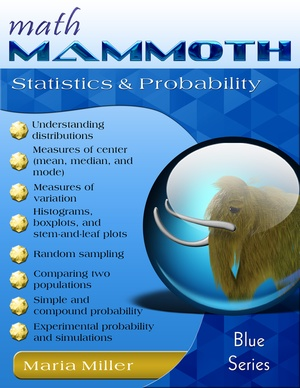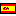^
You are here: HomeBlue Series → Statistics & Probability

# Math Mammoth Statistics & Probability136 pages

Errata

## Prices & ordering

Printed copy \$17.65

(credit cards, Google Pay, Paypal, PO)Este libro en español

Math Mammoth Statistics & Probability is a worktext covering statistics and probability topics for 6th-7th grades. Being a worktext, the book contains BOTH the instructions and exercises.

The statistics lessons in the first section are for about 6th grade level, starting with the lesson Understanding Distributions. The concept of distribution is a fundamental theme in the study of statistics. A distribution is basically how the data is distributed. The distribution can be described by its center, spread, and overall shape. The shape is read from a graph, such as a dot plot or bar graph.

Two major concepts when summarizing and analyzing distributions are its center and its variability. We study the center in the next lessons about mean, median, and mode. Students not only learn to calculate these values, but also relate the choice of measures of center to the shape of the data distribution and the type of data.

In the lesson Measures of Variation we study range, interquartile range, and mean absolute deviation. The last one takes many calculations, and the lesson gives instructions on how to calculate it using a spreadsheet program, such as Excel.

Then in the next lessons, students learn to make several different kinds of graphs: histograms, boxplots, and stem- and-leaf plots. In those lessons, students continue summarizing distributions by giving their shape, a measure of center, and a measure of variability.

The book also includes an optional statistics project, in which the student can develop investigative skills.

The next focus area of the book is random sampling. Students learn that sampling methods vary and that random sampling is likely to produce an unbiased sample — a sample that represents the population well. In the lesson Using Random Sampling, students choose several random samples from a population of 100 geometric shapes, and they see first hand that random samples can vary, sometimes a lot. Yet if the sample size is sufficiently large, or if we have several random samples, we can rather confidently conclude something about the population itself. Students will also practice making inferences about populations based on several random samples.

Another major topic is comparing two populations, either directly or by using samples from the populations. Students learn to use the overall distributions and the measures of center and variability to compare two sets of data in various ways. While some of the ways in which we compare the data are only informal, all of the concepts presented are fundamental to the use of statistics in various sciences. Students also do a project where they gather data on their own from two populations and compare them.

Probability is a new topic to the students, as it has not been studied at all in grades 1-6. However, most students have an intuitive understanding of probability based on hearing the terms “probably” and “likely,” listening to weather forecasts, and so on.

In the past, probability was only taught in high school — for example, I personally encountered it for the first time in 12th grade. However, during the 1990s and 2000s, it “crept” down the grade levels and many states required probability even in elementary school in those years. By 7th grade, students have studied fractions, ratios, and proportions, so they are ready to study probability, and also because students need an understanding of basic probability concepts in order to study statistical concepts in middle and high school.

We start with the concept of simple (classic) probability, which is defined as the ratio of the number of favorable outcomes to the number of all possible outcomes. Students calculate probabilities that involve common experiments, including flipping a coin, tossing a dice, picking marbles, and a spinner.

The lesson Probability Problems from Statistics introduces probability questions involving the phrase “at least,” which are often solved by finding the probability of the complement event. For example, it might be easier to count the number of students who got at most D+ on a test than to count the number of students who got at least C-.

In the next lesson, Experimental Probability, students conduct experiments, record the outcomes, and calculate both the theoretical and experimental probabilities of events, comparing the two. They get to roll a die hundreds of times and draw a card from a deck. The download version of this curriculum includes spreadsheet files for some of the probability simulations. You can also access those simulations online.

Next, we study compound events, which are events that involve two or more individual events. An example of this is tossing a die two times or choosing a girl, then a boy from a group of people. Students calculate the probabilities of compound events by using the complete sample space (a list of all possible outcomes). There are several ways to construct the sample space, such as by drawing a tree diagram, making a table, or sometimes simply by listing all the possible outcomes using logical thinking.

The last major topic in this book is simulations. Students design simulations to find probabilities of events. For example, we let the heads and tails of a coin represent a female or male, and toss a coin to simulate the event of choosing a person randomly. Later in the lesson, students design simulations that use random numbers. They generate those numbers either by using the free tool at Random.org/integers or a spreadsheet program on a computer.

In the last lesson of the book, Probabilities of Compound Events, we learn to calculate the probability of a compound event by multiplying the probabilities of the individual events (assuming the outcomes of the individual events are independent of each other).

## Better Yet - Bundle Deals!

*BONUS*: Buy any bundle listed above, and get the fully upgraded Soft-Pak software for FREE (includes 4 math and 2 language arts programs, complete with on screen and printable options).

## How and where to order

You can buy Math Mammoth books at:

• Here at MathMammoth.com website — simply use the "Add to cart" buttons you see on the product pages.
• Rainbow Resource carries printed copies for the Light Blue series books, plus several CDs (Light Blue and Blue series).
• At Teachers Pay Teachers you can purchase the Light Blue Series downloads, plus topical units.
• Lulu sells printed copies for most of the Math Mammoth materials (various series).

By purchasing any of the books, permission IS granted for the teacher (or parent) to reproduce this material to be used with his/her students in a teaching situation; not for commercial resale. However, you are not permitted to share the material with another teacher.

In other words, you are permitted to make copies for the students/children you are teaching, but not for other teachers' usage.

Math Mammoth books are PDF files. You will need Adobe Reader to view them, including if you use a Mac or Linux. You can try other PDF viewers, but they seem to either omit or mess up some of the images.
WAIT!

Receive my monthly collection of math tips & resources directly in your inbox — and get a FREE Math Mammoth book!You can unsubscribe at any time.

### Math Mammoth Tour

Confused about the different options? Take a virtual email tour around Math Mammoth! You'll receive:

An initial email to download your GIFT of over 400 free worksheets and sample pages from my books. Six other "TOURSTOP" emails that explain the important things and commonly asked questions concerning Math Mammoth curriculum. (Find out the differences between all these different-colored series!)

This way, you'll have time to digest the information over one or two weeks, plus an opportunity to ask me personally about the curriculum.
A monthly collection of math teaching tips & Math Mammoth updates (unsubscribe any time)### "Mini" Math Teaching Course

This is a little "virtual" 2-week course, where you will receive emails on important topics on teaching math, including:

- How to help a student who is behind
- Troubles with word problems
- Teaching multiplication tables
- Why fractions are so difficult
- The value of mistakes
- Should you use timed tests
- And more!

A GIFT of over 400 free worksheets and sample pages from my books right in the very beginning.A monthly collection of math teaching tips & Math Mammoth updates (unsubscribe any time)
Enter your email to receive math teaching tips, resources, Math Mammoth news & sales, humor, and more! I tend to send out these tips about once monthly, near the beginning of the month, but occasionally you may hear from me twice per month (and sometimes less often).• A GIFT of over 400 free worksheets and sample pages from my books.